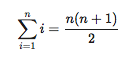# LaTeXMathJax

Summary: MathJax support for PmWiki
Prerequisites: none
Status: stable
Maintainer: Stefan Röttger (snroettg@gmail.com)
Categories: Math
Discussion: LaTeXMathJax-Talk?

## Questions answered by this recipe

How do I easily display formulas in LaTeX-style with PmWiki?

All sections are optional, you can remove those that do not apply to your recipe, and add new ones.

## Description

If you know how to typeset formulas with LaTeX, you can typeset formulas with PmWiki in the very same style.

In LaTeX formulas are typeset using the $-sign as formula delimiter. A centered formula on its own line is typeset with the double$-sign, For example, the following formula markup

$$\sum_{i=1}^{n}i = \frac{n(n+1)}{2}$$

displays as:## Installation

Latest MathJax version: LaTeXMathJax.phpΔ

Just copy the above script to your cookbook/ and add include_once("cookbook/LaTeXMathJax.php"); to your local/config.php .

## Usage

Example for in-line formula style:

The quadratic polynomial $f(t) = c_0 + c_1t + c_2t^2$ has three coefficients $c_0, c_1, c_2$.

Example for centered formula style:

$$\left( \begin{array}{c c c} n & \sum_i t_i & \sum_i t_i^2 \\ \sum_i t_i & \sum_i t_i^2 & \sum_i t_i^3 \\ \sum_i t_i^2 & \sum_i t_i^3 & \sum_i t_i^4 \end{array} \right) \left( \begin{array}{c} c_0 \\ c_1 \\ c_2 \end{array} \right) = \left( \begin{array}{c} \sum_i m_i \\ \sum_i m_i t_i \\ \sum_i m_i t_i^2 \end{array} \right)$$